# UltrasonicSensor

Friends!
Which function returns millisecond values ​​in the UltrasonicSensor service?
I need to write a script using two HC-SR04 sensors, as in this example for Arduino:
https://www.instructables.com/id/Dual-Sensor-Echo-Locator/

## Comment viewing options### Helllo alexstarter, Check the

Helllo alexstarter,

Check the UltrasonicSensor service page :

http://myrobotlab.org/service/ultrasonicsensor

At the bottom of a service page is an example script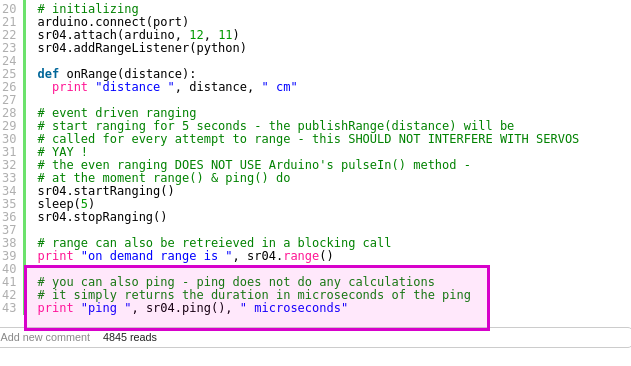### This returns me the same

This returns me the same values ​​in cm.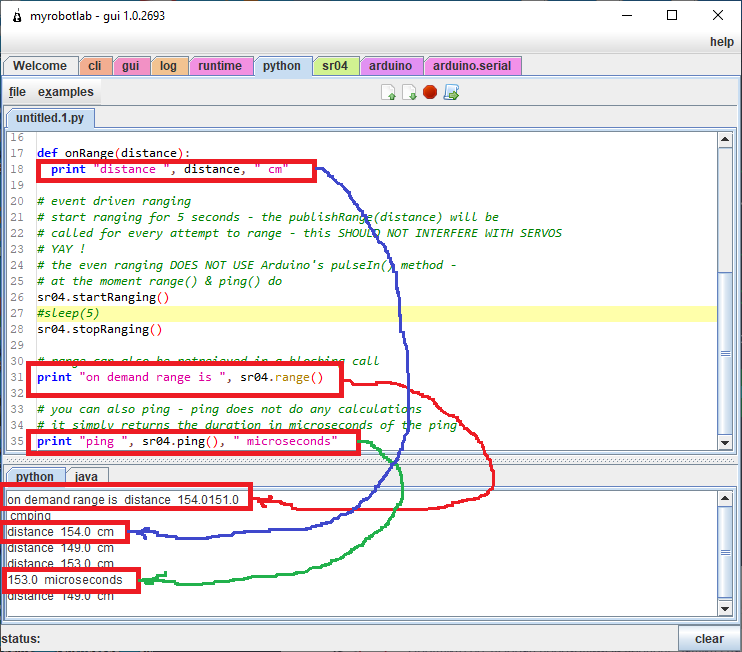### Ha !   Thanks. I looked into

Ha !   Thanks.

I looked into the details to see what was going on.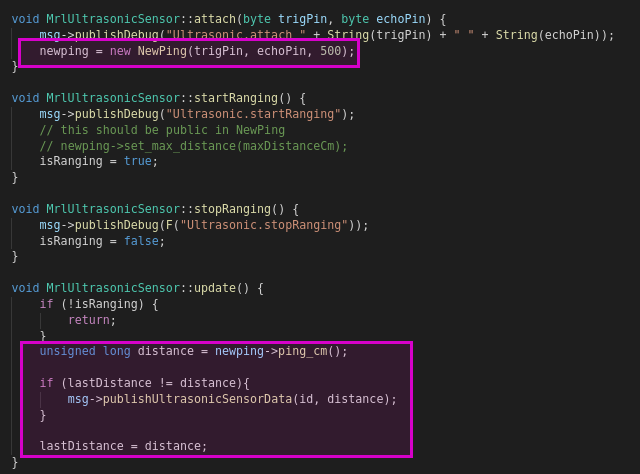NewPing was used, and instead of the time being sent back to the arduino the ping_cm() was.
The reason we switched to NewPing, is the previous Ping in Arduino blocked communication until the the time came back or there was a timeout.

I'll fix this in Nixie, for Manticore version of MRL you can just multiply it to get microseconds or milliseconds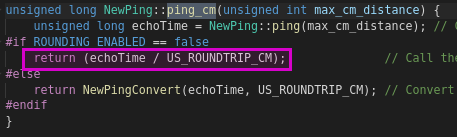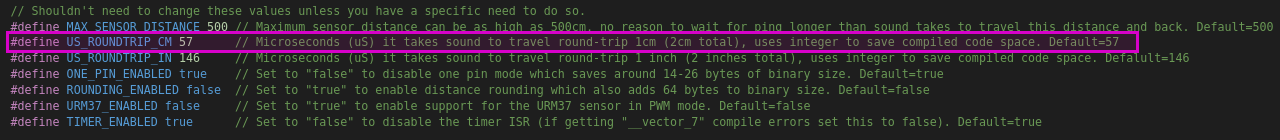Therefore echoTime = distance_cm * 57 in micro-seconds.

Hope this helps.### Thank You, GroG! I will

Thank You, GroG! I will definitely try it tomorrow.### Hi GroG! I understand that

Hi GroG!
I understand that these settings are made in MrlComm for Arduino. Please tell me which variable is responsible for the polling rate of the HC-SR04. And yet, I need to do a synchronous launch of two HC-SR04. Because one of them will only listen.### The details are in

The details are in MrlUltrasonicSensor.cpp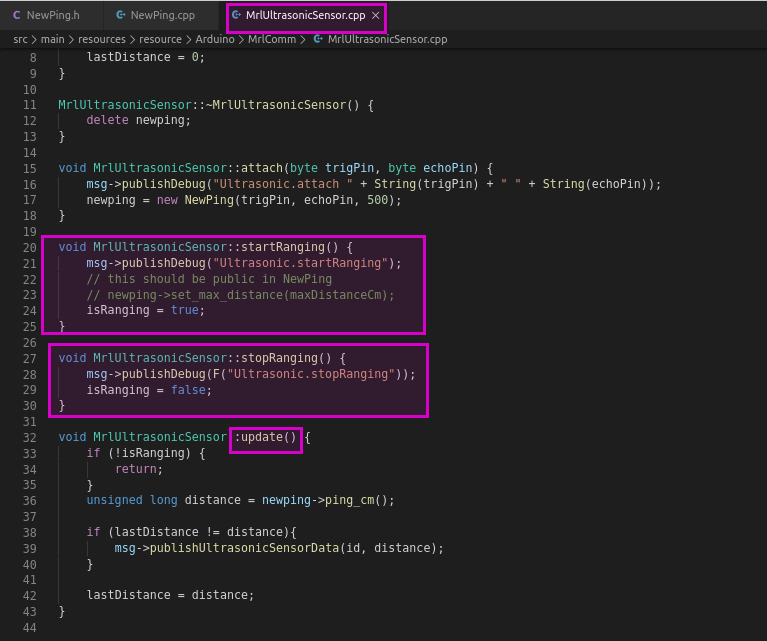Within MrlComm devices - update() is called in a processing loop which has a high as possible frequency rate.  MrlUltrasonicSensor.cpp uses NewPing to process the ping.  calling ping_cm() before the echo has processed, is a noop I believe.

If you want to synchronize multiple Sensors I would suggest that you NOT do it in MrlComm.  Rather use the sensor startRanging() stopRanging() in Python.

e.g.

s1 = Runtime.start("s1","UltrasonicSensor")
s2 = Runtime.start("s2","UltrasonicSensor")
current_sensor = "s1"

def onRange(rang):
.. do something ..

while (True):

if current_sensor == "s1"
s2.stopRanging()
s1.startRanging()
sleep(3)
current_sensor = "s2"
else:
s1.stopRanging()
s2.startRanging()
sleep(3)
current_sensor = "s1"

this alternates 3 seconds for each sensor### Oh, GroG ! It's great that

Oh, GroG ! It's great that you took the time to give me an example. Thanks! I will definitely try it!GroG.
Your example synchronizes the sensors alternately.
I need to request both sensors at the same time.### Hmm... I would think "at the

Hmm... I would think "at the same time"  is not a good idea -
If you have the sensor placed anywhere near one another, you'll start getting interference from one to the other.

I'd recommend you just reduce the sleep time between the sensors but keep them alternating.  You can probably reduce the time so much the "appear" to be working simultaneously.### Thank GroG I understood

Thank GroG
I need to request sensors alternately. And then mathematically determine the movement of an object from side to side.
This is necessary so that the platform for the robot can follow me.
I slightly modified and printed out the proposed Marcus - Mecanum_wheels_for_Robyn_inmoov.
Now I need to learn how to manage it.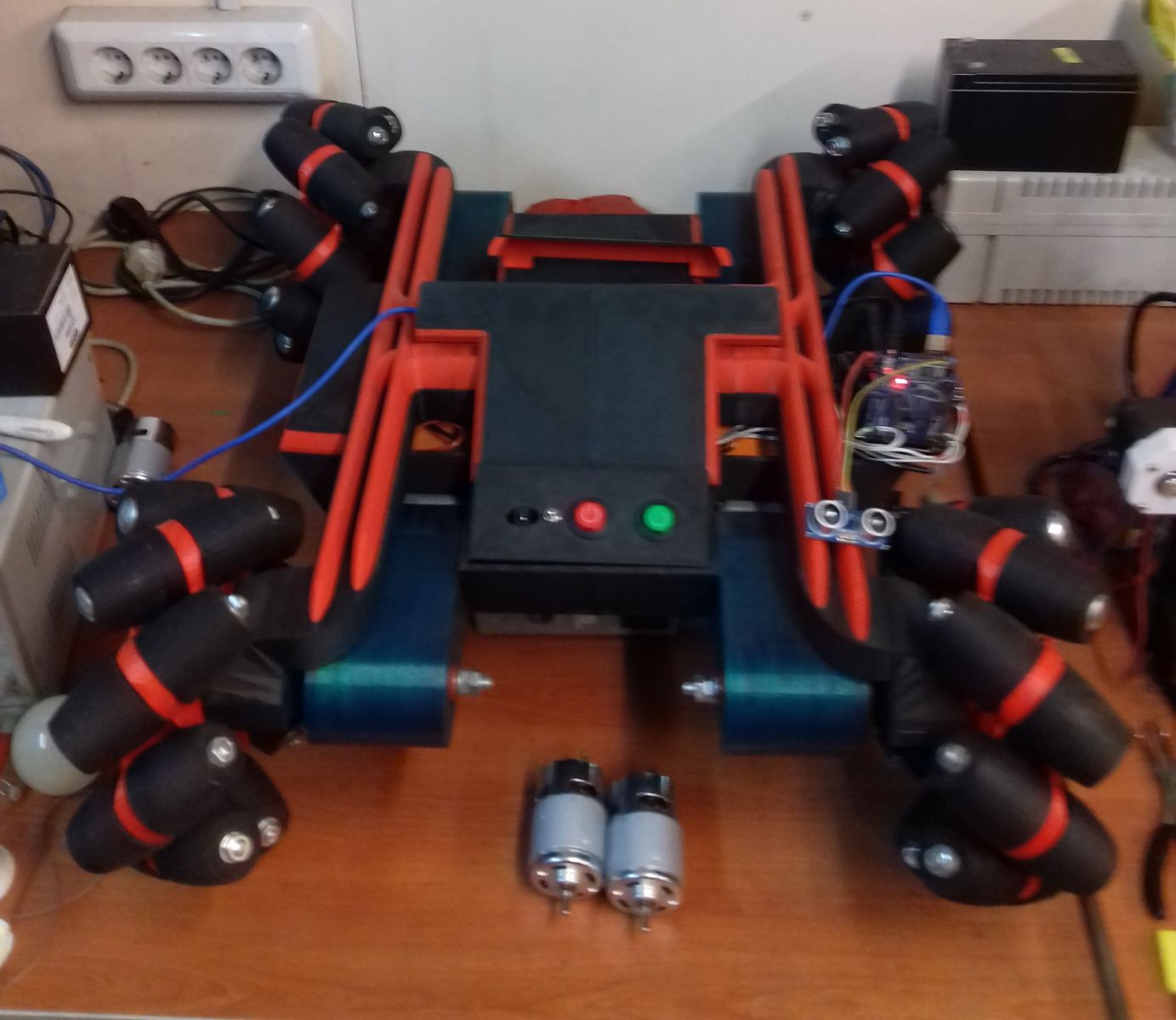### Looks Great Alextstarter !

Looks Great Alextstarter !### Hi GroG!  I am new to

Hi GroG!

I am new to Python. Therefore, my scenario will seem wrong to you. The script needs optimization, I know that. But even in this form, it works.

port = "COM3"
PlatMax = 60
PlatMin = 35
Delta = 60 # разница между датчиками для разворота
Sig = .02 # приращение скорости для плавного пуска и стопа
flag = 1 # флаг, что мы сдесь были

SPEED = 10
arduino = runtime.start("arduino","Arduino")
python = Runtime.start("python","Python")
motorFR = runtime.start("motorFR","MotorDualPwm")
motorFL = runtime.start("motorFL","MotorDualPwm")
motorRR = runtime.start("motorRR","MotorDualPwm")
motorRL = runtime.start("motorRL","MotorDualPwm")
sr04_A = Runtime.start("sr04_A", "UltrasonicSensor")
sr04_B = Runtime.start("sr04_B", "UltrasonicSensor")
current_sensor = "sr04_A"
pin1 = Runtime.start('pin1','Relay')
pin2 = Runtime.start('pin2','Relay')
pin3 = Runtime.start('pin3','Relay')
pin4 = Runtime.start('pin4','Relay')

# initializing
arduino.connect(port)
sr04_A.attach(arduino, 50, 52)
sr04_B.attach(arduino, 46, 48)

motorFR.setPwmPins(3,2)
motorFR.attach(arduino)
motorFL.setPwmPins(5,4)
motorFL.attach(arduino)
motorRR.setPwmPins(7,6)
motorRR.attach(arduino)
motorRL.setPwmPins(9,8)
motorRL.attach(arduino)
pin1.arduino = arduino
pin1.pin = 22
pin1.off()
pin2.arduino = arduino
pin2.pin = 24
pin2.off()
pin3.arduino = arduino
pin3.pin = 26
pin3.off()
pin4.arduino = arduino
pin4.pin = 28
pin4.off()

def STOP(speed,routeFR,routeFL,routeRR,routeRL):
global flag
for x in range(0,abs(speed) + 1):

if routeFR < 0: pwmFR = (abs(speed) - x) * -Sig
if routeFR > 0: pwmFR = (abs(speed) - x) * Sig

if routeFL < 0: pwmFL = (abs(speed) - x) * -Sig
if routeFL > 0: pwmFL = (abs(speed) - x) * Sig

if routeRR < 0: pwmRR = (abs(speed) - x) * -Sig
if routeRR > 0: pwmRR = (abs(speed) - x) * Sig

if routeRL < 0: pwmRL = (abs(speed) - x) * -Sig
if routeRL > 0: pwmRL = (abs(speed) - x) * Sig
print('stop ',pwmFR,' ',pwmFL,' ',pwmRR,' ',pwmRL)
motorFR.move(pwmFR); motorFL.move(pwmFL); motorRR.move(pwmRR); motorRL.move(pwmRL)
flag = 1

def UP(speed):
global flag
for x in range(0,abs(speed) + 1):
pwmFR = x * Sig
pwmFL = x * Sig
pwmRR = x * Sig
pwmRL = x * Sig
print('UP ',pwmFR,' ',pwmFL,' ',pwmRR,' ',pwmRL)
motorFR.move(pwmFR); motorFL.move(pwmFL); motorRR.move(pwmRR); motorRL.move(pwmRL)
flag = 3

def DOWN(speed):
global flag
for x in range(0,abs(speed) + 1):
pwmFR = x * -Sig
pwmFL = x * -Sig
pwmRR = x * -Sig
pwmRL = x * -Sig
print('DOWN ',pwmFR,' ',pwmFL,' ',pwmRR,' ',pwmRL)
motorFR.move(pwmFR); motorFL.move(pwmFL); motorRR.move(pwmRR); motorRL.move(pwmRL)
flag = 2

def RIGHT_TURN(speed):
global flag
for x in range(0,abs(speed) + 1):
pwmFR = x * -Sig
pwmFL = x * Sig
pwmRR = x * -Sig
pwmRL = x * Sig
print('RIGHT_TURN ',pwmFR,' ',pwmFL,' ',pwmRR,' ',pwmRL)
motorFR.move(pwmFR); motorFL.move(pwmFL); motorRR.move(pwmRR); motorRL.move(pwmRL)
flag = 5

def LEFT_TURN(speed):
global flag
for x in range(0,abs(speed) + 1):
pwmFR = x * Sig
pwmFL = x * -Sig
pwmRR = x * Sig
pwmRL = x * -Sig
print('LEFT_TURN ',pwmFR,' ',pwmFL,' ',pwmRR,' ',pwmRL)
motorFR.move(pwmFR); motorFL.move(pwmFL); motorRR.move(pwmRR); motorRL.move(pwmRL)
flag = 4

def onRange(rang):
if current_sensor == "sr04_A": distance_A = sr04_A.range()
if current_sensor == "sr04_B": distance_B = sr04_B.range()
distance = (distance_A + distance_B)/2
print ('distance  ' , distance,'A  ' ,distance_A,'B  ' ,distance_B)

if ((distance > PlatMin) and (distance < PlatMax) and flag != 1):
if flag == 2:
STOP(SPEED,-1,-1,-1,-1)
elif flag == 3:
STOP((SPEED),1,1,1,1)
elif flag == 4:
STOP((SPEED),1,-1,1,-1)
elif flag == 5:
STOP((SPEED),-1,1,-1,1)
elif (((distance_A - distance_B) < 0) and (abs(distance_A - distance_B) > Delta) and flag != 4):
if flag == 2: STOP((SPEED),1,1,1,1)
elif flag == 3: STOP((SPEED),1,1,1,1)
elif flag == 5: STOP((SPEED),-1,1,-1,1)
LEFT_TURN(SPEED)
elif (((distance_A - distance_B) > 0) and (abs(distance_A - distance_B) > Delta) and flag != 5):
if flag == 2: STOP((SPEED),1,1,1,1)
elif flag == 3: STOP((SPEED),1,1,1,1)
elif flag == 4: STOP((SPEED),1,-1,1,-1)
RIGHT_TURN(SPEED)
elif (((distance_A - distance_B) > 0) and (abs(distance_A - distance_B) > Delta) or
((distance_A - distance_B) < 0) and (abs(distance_A - distance_B) > Delta)):
return
elif distance < PlatMin and flag != 2:
if flag == 3: STOP((SPEED),1,1,1,1)
elif flag == 4: STOP((SPEED),1,-1,1,-1)
elif flag == 5: STOP((SPEED),-1,1,-1,1)
DOWN(SPEED)
elif distance > PlatMax and flag != 3:
if flag == 2: STOP((SPEED),1,1,1,1)
elif flag == 4: STOP((SPEED),1,-1,1,-1)
elif flag == 5: STOP((SPEED),-1,1,-1,1)
UP(SPEED)

while (True):
#print (current_sensor)
if current_sensor == "sr04_A":
sr04_B.stopRanging()
sr04_A.startRanging()
sleep(0.25)
current_sensor = "sr04_B"
else:
sr04_A.stopRanging()
sr04_B.startRanging()
sleep(0.25)
current_sensor = "sr04_A"### Tell me how can I post a

Tell me how can I post a video?

https://vk.com/wall187347478_98### I posted your video here

I posted your video here - http://myrobotlab.org/content/ultrasonic-sensors-and-mecanum-wheel-platform

Its pretty easy, you just need the embed code from your video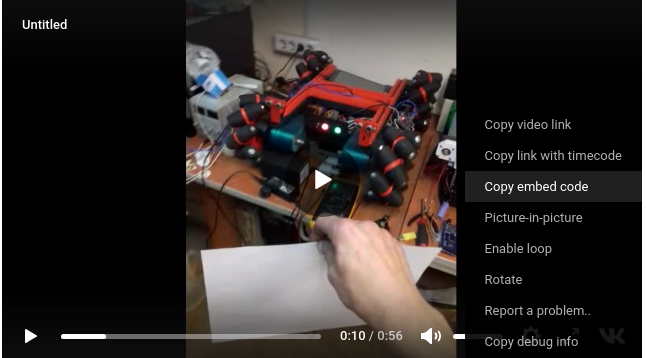press this button and copy / paste the embed code in it### UltrasonicSensor

Hello Grog!

I cannot start two UltrasonicSensors at once on my InMoov.
In the tutorial, on the site https://inmoov.fr/howto-ultrasonic/ it is stated like this:
Open InMoov/config/service_I_UltraSonicSensor.config and setup like below.

`[MAIN]`

```ultraSonicRightActivated=True ultraSonicRightArduino=right trigRightPin=64 echoRightPin=63```

```ultraSonicLeftActivated=False ultraSonicLeftArduino=left trigLeftPin=64 echoLeftPin=63```

But that doesn't work.

I think there is a problem in the file

C:\mrl\InMoov\services    I_UltraSonicSensor.py

# -- coding: utf-8 --
# ##############################################################################
#                 ultra Sonic Sensor FILE
# ##############################################################################

#parse config
ThisServicePart=RuningFolder+'config/service_'+os.path.basename(inspect.stack()).replace('.py','')

CheckFileExist(ThisServicePart)
ThisServicePartConfig = ConfigParser.ConfigParser()
trigPin=ThisServicePartConfig.getint('MAIN', 'trigPin')
echoPin=ThisServicePartConfig.getint('MAIN', 'echoPin')
ultraSonicSensorArduino=ThisServicePartConfig.get('MAIN', 'ultraSonicSensorArduino')
ultraSonicSensorActivated=ThisServicePartConfig.getboolean('MAIN', 'ultraSonicSensorActivated')

if ultraSonicSensorActivated:
ultrasonicSensor = Runtime.start("ultrasonicSensor", "UltrasonicSensor")

try:
ultraSonicSensorArduino=eval(ThisServicePartConfig.get('MAIN', 'ultraSonicSensorArduino'))
ultrasonicSensor.attach(ultraSonicSensorArduino, trigPin, echoPin)
i01.ultrasonicSensor=ultrasonicSensor
talkEvent(lang_startingUltraSonic)
# range can also be retreieved in a blocking call
print "ultrasonicSensor test is ", i01.getUltrasonicSensorDistance()
except: